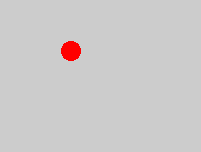# Animation

Animation is one of the primary uses of the `Runnable` interface. To animate objects in Java, you create a thread that calculates successive frames and then calls `repaint()` to paint the screen. You could just stick an infinite loop in your `paint()` method, but that is quite dangerous, especially on non-preemptive systems like the Mac. It also doesn't provide any support for timing.Let's begin with a simple animation that bounces a red ball around in a box. The red ball will just be a big red circle. Its coordinates will be stored in a `java.awt.Rectangle` field called ball. The `paint()` method will do nothing but look at this field and then draw it.

The `run()` method of this applet is where the action will take place. Here you'll increment the coordinates of the ball, then check to see if the ball has moved to the edge of the visible area. If it has reverse the direction of the ball. The applet's really quite simple. Here it is:

``````import java.awt.*;
import java.applet.*;

public class Bounce extends Applet implements Runnable {

private Rectangle r;
private int deltaX = 1;
private int deltaY = 1;

public void init () {
r = new Rectangle( 30, 40, 20, 20);
t.start();
}

public void paint (Graphics g) {
g.setColor(Color.red);
g.fillOval(r.x, r.y, r.width, r.height);
}

public void run() {

while (true) {  // infinite loop
r.x += deltaX;
r.y += deltaY;
if (r.x >= size().width || r.x < 0) deltaX *= -1;
if (r.y >= size().height || r.y < 0) deltaY *= -1;
this.repaint();
}

}

}``````

Copyright 1997-1999, 2002 Elliotte Rusty Harold
elharo@metalab.unc.edu